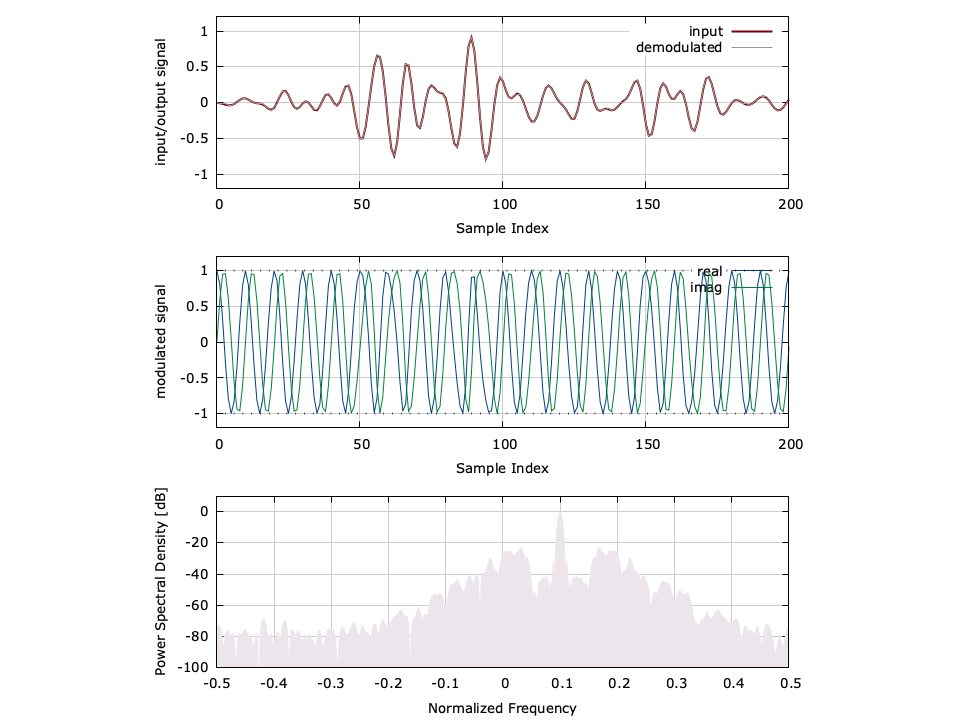# Analog Frequency Modulator/Demodulator (freqmod, freqdem)

The freqmod and freqdem objects implement the analog frequency modulation (FM) modulator and demodulator, respectively, in liquid. Given an input message signal $$-1 \le m(t) \le 1$$ , the transmitted signal at complex baseband is

$$s(t) = \exp\left\{ j 2 \pi k_f \int_{0}^{t}{ m(\tau)d\tau } \right\}$$

where $$k_f$$ is the modulation factor. For a discrete-sampled time series, the transmitted signal at time index $$k$$ is simply

$$s[k] = \exp\left\{ j 2 \pi k_f \sum_{j=0}^{k-1}{m[j]} \right\}$$

The modulation index governs the relative bandwidth of the signal. For a received time series $$\vec{r} = \left\{r,r,\ldots,r[k] \right\}$$ , demodulation is performed simply by computing the instantaneous phase difference between adjacent samples, viz

$$\hat{m}[k] = \frac{1}{2 \pi k_f} \arg\left\{ r^*[k-1] r[k] \right\}$$

This provides an estimate of the input message signal, represented as $$\hat{m}$$ in [eqn-modem-freqmod-rx-discrete] above. An example of the freqmod and freqdem object outputs can be found in [fig-modem-freqmodem] , below.Figure [fig-modem-freqmodem]. freqmodem demonstration modulating an audio signal with a modulation index of $$k_f=0.1$$ and a relative carrier frequency $$f_c/F_s = 0.1$$ .

An example of the interface is listed below.


#include <liquid/liquid.h>

int main() {
float kf = 0.1f;        // modulation factor

// create modulator/demodulator objects
freqmod fmod = freqmod_create(kf);
freqdem fdem = freqdem_create(kf);

float m;                // input message
float complex s;        // modulated signal
float y;                // output/demodulated message

// repeat as necessary
{
// modulate signal
freqmod_modulate(fmod, m, &s);

// demodulate signal
freqdem_demodulate(fdem, s, &y);
}

// clean up objects
freqmod_destroy(fmod);
freqdem_destroy(fdem);
}


A more detailed example can be found in examples/freqmodem_example.c located under the main liquid project source directory.

## Interface

Listed below is the full interface to the freqmod object for analog frequency modulation.

• freqmod_create(kf) creates and returns an freqmod object with a modulation factor $$k_f$$ .
• freqmod_destroy(q) destroys an freqmod object, freeing all internally-allocated memory.
• freqmod_print(q) prints the internal state of the freqmod object.
• freqmod_reset(q) resets the state of the freqmod object.
• freqmod_modulate(q,m,*s) modulates the input sample $$m$$ storing the single output sample to $$s$$ .
• freqmod_modulate_block(q,*m,n,*s) modulates $$n$$ input samples of $$m$$ and stores the output to the array $$s$$ of equal length.

The interface for the demodulator ( freqdem ) is shown here:

• freqdem_create(kf) creates and returns an freqdem object with a modulation factor $$k_f$$ .
• freqdem_destroy(q) destroys an freqdem object, freeing all internally-allocated memory.
• freqdem_print(q) prints the internal state of the freqdem object.
• freqdem_reset(q) resets the state of the freqdem object.
• freqdem_demodulate(q,s,*m) demodulates the input sample $$s$$ storing the output estimate of the message signal to the single sample $$m$$ .
• freqdem_demodulate_block(q,*s,n,*m) demodulates $$n$$ input samples of $$s$$ and stores the output to the array $$m$$ of equal length.